• Call Now

1800-102-2727•

# Kirchoff's Law: Explanation, Formula, Wien's Displacement Law, Examples

Different objects emit radiation of different wavelengths at different temperatures. Everyone has seen the colour of the flame for different kinds of fire like matchstick burning, kitchen stove etc. Basically it depends upon their temperature but to know about what makes them look different to human eyes, we need to know about some laws related to radiation.

• Electromagnetic Spectrum
• Distribution of energy in the spectrum of a black body
• Wien’s Displacement Law
• Practice problems
• FAQs

Kirchhoff's law of radiation states that the ratio of emissive power to absorptive power is the same for all bodies at a given temperature and is equal to the emissive power of a blackbody at that temperature. The good absorbers of radiation are also good emitters.

Emissive power: It is defined as the amount of energy radiated per unit area per unit time. The expression of this is given as follows: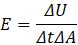Where E is the energy radiated per unit time per unit area.

The unit of emissive power is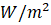and the dimensions are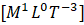.

## Absorptive power

It is a fraction of the incident radiation that is absorbed by the body. Mathematically,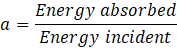Since it is the ratio of similar quantities, it has no units and dimensions.

For an ideal blackbody, absorptive power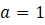Absorptive power of any body is given by,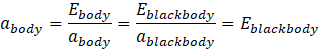Since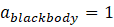## Electromagnetic Spectrum

From the whole electromagnetic spectrum, we receive infrared waves, visible rays, and ultraviolet rays of the wavelengths that lie in the range of 7.8 × 10-7 m to 4 × 10-4 at the earth. Therefore, they are collectively known as thermal radiation.The wavelengths (𝜆) of the radiations emitted from a body at a particular temperature are plotted against the intensity of the radiations (I) as shown in the graph. The distribution of the emitted energy from a body as a function of wavelength changing with respect to temperature can be deduced as follows:(i) The general shape of the curve is the same for all the temperatures.

(ii) As the temperature of the body increases, the value of the most probable wavelength (𝜆max) in the spectrum shifts or displaces towards the shorter wavelength. Thus, the plot shifts towards the left.

(iii) A high-temperature body emits a shorter wavelength (𝜆max), and a low-temperature body emits a higher wavelength (𝜆max).

Sun as a black body

The temperature of the Sun is very high. Therefore, it emits all the possible radiations, and it is an example of the black body.

## Distribution of energy in the spectrum of a black body

A perfect black body emits radiation of all the possible wavelengths.

• At a given temperature, the energy is not uniformly distributed among the different wavelengths.
• At a given temperature, the intensity of the heat radiation increases with an increase in the wavelength. The heat radiation is maximum at a particular wavelength and with further increase in the wavelength, it decreases.The area under the curve represents the total intensity of radiation at a particular temperature.

The area under the curve is given as follows: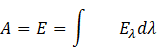## Wien’s Displacement LawFrom the intensity (I) vs wavelength (λ) plot for a particular radiation emitted from a body, the following can be deduced.

• The thermal radiation emitted by a body is a bunch of waves of different wavelengths.
• A small range of wavelengths has a significant contribution to the total radiation.
• As the temperature of the body is increased, the value of the most probable wavelength (λm) in the spectrum shifts towards the shorter wavelength region. Thus, the plot shifts towards the left retaining the shape and is named as ‘displacement law’.

Wien’s displacement law states that the wavelength of the highest intensity (most probable) is inversely proportional to the absolute temperature of the emitting body. Its mathematical expression is given as follows: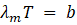Where b is a constant known as ‘Wien’s constant’ and its numerical value is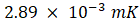## Practice problems

Q. A black body is at rest at a temperature of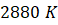. The energy of radiation emitted by this object with wavelength between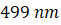and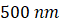is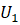, between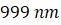and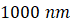is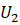and between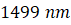and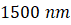is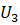. The Wien's constant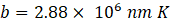. Then choose the correct statement:

1.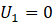2.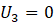3.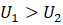4.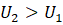A. Given, temperature of black body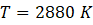From Wien's law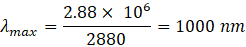Energy distribution with wavelength is as shown in the figure.

From the figure it is clear thatis corresponding to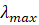, thereforeQ. Choose the correct option regarding the following statements:

Statement 1 : Radiations of longer wavelengths are predominant at lower temperature.

Statement 2 : The wavelength corresponding to maximum emission of radiation shifts from longer wavelength to shorter wavelength as the temperature increases.

1. Statement 1 is true, statement 2 is false
2. Statement 1 is false, statement 2 is true
3. Both Statement 1 and statement 2 are true
4. Both Statement 1 and statement 2 are false

A. (c)

According to Wien's Displacement Law: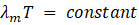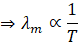From the above distribution, we can infer that, as the temperature increases, peak wavelength decreases. Therefore, radiations of longer wavelength are predominant at lower temperature.

Q. The adjoining diagram shows the spectral energy density distribution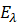of a black body at two different temperatures. If the areas under the curves are in the ratio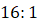, find the value of temperature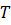.

A.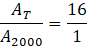Area under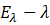curve represents the emissive power of body

Emissive power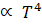Hence, area undercurve curve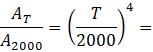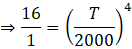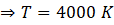Q. If between wavelength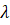and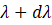,and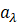are the emissive and absorptive powers of a body and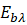is the emissive power of a perfectly black body, then according to Kirchhoff's law, which of the following is true?

1.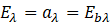2.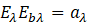3.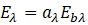4.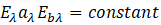A. According to Kirchhoff's law, the ratio of emissive power to absorptive power is same for all bodies and is equal to the emissive power of a perfectly black body at that wavelength i.e.

For a particular wavelength,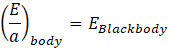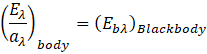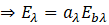## FAQs

Q. Which of the following is correct regarding a perfectly black body?

1. Emissivity of perfectly black body is equal to 1
2. Emissivity of perfectly black body is greater than 1
3. Emissivity of perfectly black body lies between 0 and 1
4. Emissivity of perfectly black body is equal to zero

A. A good absorber is a good emitter. As the perfectly black body absorbs all the radiation, it can also emit at all possible wavelengths. Therefore, the emissivity of a perfectly black body is equal to 1.

Q. The plots for intensity versus wavelength for three black bodies at temperatures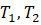and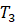respectively are shown in figure. Their temperatures are such that1.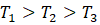2.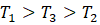3.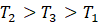4.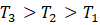A. According to Wien's displacement law,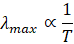Or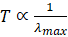From figure, it is evident that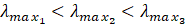Therefore, we can conclude that,Q. What is the unit of absorptive power?
A. Since it is the ratio of energy absorbed per unit area to the energy incident per unit area, absorptive power is unitless.

Q.  The flame of a peach-scented candle has a yellowish colour and the flame of a Bunsen’s burner in a chemistry lab has a bluish colour. Which flame has a higher temperature?

A. Since the wavelength of the blue flame is more than that of yellow flame, therefore as per Wien's law, temperature is inversely proportional to its wavelength at its peak intensity. Therefore, the temperature of the bluish flame of a bunsen burner will be more than that of a peach scented candle.Talk to our expert
Resend OTP Timer =
By submitting up, I agree to receive all the Whatsapp communication on my registered number and Aakash terms and conditions and privacy policy